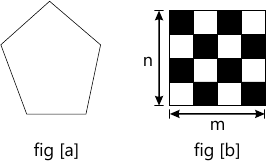MORE IN Computer Graphics
MU Computer Engineering (Semester 4)
Computer Graphics
December 2012
Total marks: --
Total time: --
INSTRUCTIONS
(1) Assume appropriate data and state your reasons
(2) Marks are given to the right of every question
(3) Draw neat diagrams wherever necessary

1 (a) Illustrate inside outside test.
5 M
1 (b) Explain 3D display methods.
5 M
1 (c) What is z-buffer algorithm?
5 M
1 (d) Write a note on Fractals.
5 M

2 (a) What is a display file structure? Also explain the need for display file interpreter.
10 M
2 (b) Wrte a pseudo-code to implement boundary fill and flood fill algorithm using 4 connected method.
10 M

3 (a) Write an algorithm to fill polygon in fig (a) with pattern given in fig(b) of size m×n.10 M
3 (b) Derive Bresenham's line drawing algorithm for lines with slope < 1.
5 M
3 (c) Write different applications of computer graphics.
5 M

4 (a) What do you mean by segment? What are the various attributes in segment table? State which operations can be on segments and explain the same.
10 M
4 (b) Derive the steps required to perform 3D rotation about arbitrary axis.
10 M

5 (a) Find the clipping Co-ordinates to clip the line segment PIP2 against the window ABCD using Cohen Sutherland line clipping algorithm.
P1(10, 30) P2(80, 90)
window ABCD A(20, 20) B(90, 20) C(90, 70) D(20, 70)
10 M
5 (b) What do you understand by terms parallel and perspective projection? Also explain depth cueing.
10 M

10 M
6 (b) Explain Bezier curves and Bezier surface with equations. Explain properties of Bezier curve.
10 M

Write short notes on (any four):-
7 (a) Animation
5 M
7 (b) Dithering techniques
5 M
7 (c) Warnock's algorithm
5 M
7 (d) Half toning
5 M
7 (e) Raster techniques
5 M

More question papers from Computer Graphics